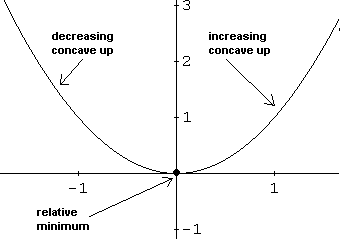# First, Second Derivatives and Graphs of Functions

A tutorial on how to use the first and second derivatives, in calculus, to study the properties of the graphs of functions.

## Theorems

To graph functions in calculus we first review several theorem. 3 theorems have been used to find maxima and minima using first and second derivatives and they will be used to graph functions. We need 2 more theorems to be able to study the graphs of functions using first and second derivatives.
Theorem 4: If f is differentiable on an interval (I1 , I2) and differentiable on [I1 , I2] and
4.a - If f ' (x) > 0 on (I1 , I2) , then f is increasing on [I1 , I2].
4.b - If f ' (x) < 0 on (I1 , I2) , then f is decreasing on [I1 , I2].
4.c - If f ' (x) = 0 on (I1 , I2) , then f is constant on [I1 , I2].
Theorem 5: If f is twice differentiable on an interval (I1 , I2) and
5.a - If f '' (x) > 0 on (I1 , I2) , then f has concavity up on [I1 , I2].
5.b - If f ' (x) < 0 on (I1 , I2) , then f is concavity down [I1 , I2].

## Examples with Detailed Solutions

We will present examples of graphing functions using the theorems in "using first and second derivatives" and theorems 4 and 5 above.

### Example 1

Use first and second derivative theorems to graph function f defined by
f(x) = x 2

Solution to Example 1.
step 1: Find the first derivative, any stationary points and the sign of f ' (x) to find intervals where f increases or decreases.
f ' (x) = 2x
The stationary points are solutions to:
f ' (x) = 2x = 0 , which gives x = 0.
The sign of f ' (x) is given in the table below. f ' (x) is negative on (-∞ , 0) f decreases on this interval. f ' (x) is positive on (0 , ∞) f increases on this interval. Also according to theorem 2(part a) "using first and second derivatives", f has a minimum at x = 0.step 2: Find the second derivative, its signs and any information about concavity.
f ''(x) = 2 and is always positive (this confirms the fact that f has a minimum value at x = 0 since f ''(0) = 2, theorem 3(part a)), the graph of f will be concave up on (-∞ , +∞) according to theorem 5(part a) above.
step 3: Find any x and y intercepts and extrema.
y intercept = f(0) = 0.
x intercepts are found by solving f(x) = x
2 = 0. x intercept = 0.
From the signs of f ' and f'', there is a minimum at x = 0 which gives the minimum point at (0 , 0).
step 4: Put all information in a table and graph f.
Also as x becomes very large (+∞) or veyy small (-∞) , f(x) = x
2 becomes very large.
See table above and graph below.### Example 2

Use first and second derivative theorems to graph function f defined by
f(x) = x 3 - 4x 2 + 4x

Solution to Example 2.
step 1: f ' (x) = 3x 2 - 8x + 4.
Solve 3x
2 - 8x + 4 = 0
solutions are: x = 2 and x = 2/3, see table of sign below that also shows interval of increase/decrease and maximum and minimum points.
step 2: f '' (x) = 6x - 8.
Solve 6x - 8 = 0 ; solution is x = 4/3 inflection point: where concavity changes. See sign and concavity in table below.
step 3: y intercept = f(0) = 0. x intercepts solve x 3 - 4x 2 + 4x = 0.
factor x out x(x
2 - 4x + 4) = 0 and solve the quadratic equation x 2 - 4x + 4 = (x - 2) 2 = 0 to find solutions: x = 0, x = 2 of multiplicity 2.
Also as x becomes very large (+∞) , f(x) = x
3 - 4x 2 + 4x becomes very large (+∞). As x becomes very small (-∞), f(x) becomes very small (-∞).
step 4: The table and the graph are shown below.More references on calculus problems